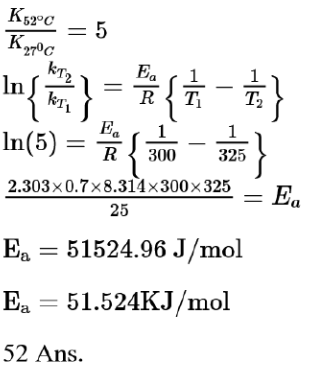# The rate constant of a reaction increases by five times on increase in temperature from

Question:

The rate constant of a reaction increases by five times on increase in temperature from $27^{\circ} \mathrm{C}$ to $52^{\circ} \mathrm{C}$. The value of activation energy in $\mathrm{kJmol}^{-1}$ is ___________(Rounded off to the nearest integer)

$\left[\mathrm{R}=8.314 \mathrm{~J} \mathrm{~K}^{-1} \mathrm{~mol}^{-1}\right]$

Solution:

(52)